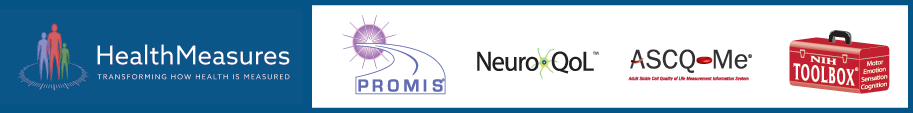# Item Response Theory (IRT)

## Many instruments in HealthMeasures are based on item response theory (IRT). IRT is a family of mathematical models that assumes that responses on a set of items or questions are related to an unmeasured “trait”. An example of such a trait may be physical function. IRT models assume a person’s level on physical function (e.g., high vs. low) will predict that person’s probability of endorsing each specific item.

#### Parameters and Calibration

When applying IRT, instrument developers assign unique values to each item based on how likely people with different levels of the measured trait are to endorse an item. Once these item values (“parameters”) are estimated (“calibrated”) for each item in a questionnaire or item bank, the parameters can be used to score any new response data from any subset of items. To learn more about parameters, please see part 4 of Karon Cook’s video series “Understanding Item Parameters: Difficulty and Discrimination”.

An IRT model estimates how individuals with given trait levels will respond to items with specified characteristics (called parameters). Examples of parameters include item difficulty and item discrimination. Models are classified by:

• The number of item parameters estimated,
• The number of response options (two vs. more than two), and
• The mathematical relationships assumed among item parameters (how the model is parameterized).

IRT models for items that have only two possible response options are called dichotomous response models.

IRT models for items that have more than two possible response options are called polytomous response models.

#### IRT vs. Classical Test Theory

IRT is often called ‘‘modern psychometric theory’’ to distinguish it from “classical test theory” (CTT).

Scores based on CTT require that participants respond to every item of a measure or that missing responses be imputed. To get a score using CTT you might:

• Sum item response scores
• Calculate the mean of the response scores
• Use some other arithmetic equation to calculate scale score based on item scores

IRT-based scores are estimated based on a probability model that answers this question:

• Given what is known about the items a person responded to and the pattern of the person’s response, what is the most likely level of the trait (domain) being measured?

#### Types of IRT Models used in HealthMeasures

The two IRT models used in health measures are the 1-parameter logistic model and the graded response model.

#### Thresholds vs. Intercepts

The graded response model has two parameters: a slope [a], and either a threshold [b], or an intercept [c]. The threshold is historically most common, as it represents the score where there is a 50% probability of choosing that response. However, most current IRT software use intercepts (which do not have the same interpretability as thresholds). Intercepts are necessary for fitting multidimensional models. Unidimensional models, such as those used by HealthMeasures, can be fit with either parameterization, and can be readily transformed (b=-c/a or c=-a*b).

Computer adaptive tests (CATs) are all but impossible without IRT. Many CATs are available through HealthMeasures.